# Shikaku

﻿﻿﻿﻿﻿﻿﻿﻿﻿﻿﻿﻿﻿﻿﻿﻿﻿﻿﻿﻿﻿
Translate this site.

Shikaku (also known as Rectangles) is a logic puzzle with simple rules and challenging solutions.

The rules are simple. You have to divide the grid into rectangular and square pieces such that each piece contains exactly one number, and that number represents the area of the rectangle.

Click and drag to draw a rectangle. To remove lines use the right mouse button or hold the Ctrl or the Shift key.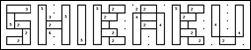Q. How to mark a rectangle?
A. It is enough to start marking the rectangle at the middle of the start square and release the mouse at the somewhere inside the opposite square. Something like this:Q. How to remove a line:
A. You should surround the lines you want removed with the red marking tool. That happens when you use the right mouse button: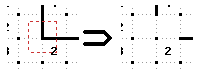Q. How to solve a puzzle:
A. Here is an example of how a puzzle is solved: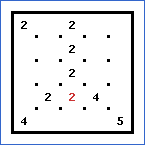First we notice that the only rectangle with 2 squares that can fit the red 2 is this one:Then notice the red 4 and 5 in the bottom corners. Their rectangles can be only the following:The red 2 in the top-left corner is analogical to the first one.  The squares below the bottom red 2 and 4 should definitely be part of their rectangles since there cannot be a rectangle without a number. So this is the result: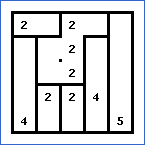It is quite easy to finish the puzzle from this point: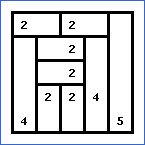www.puzzle-shikaku.com